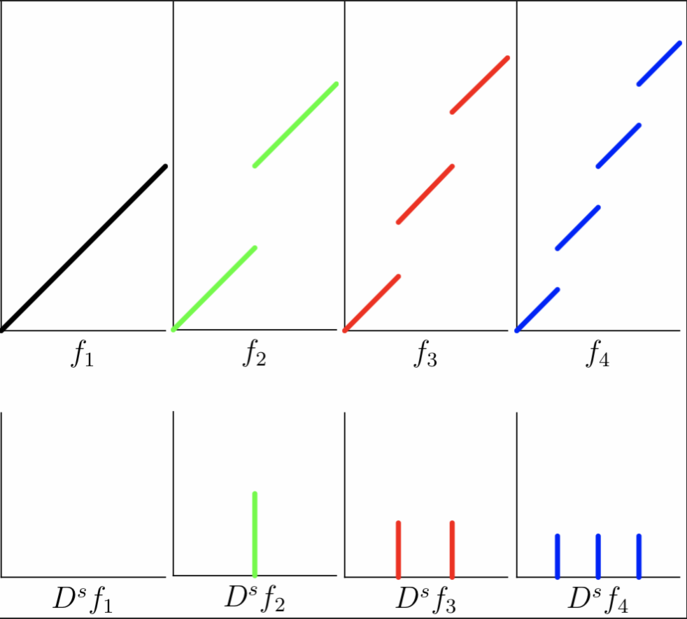# Structured deformations of continua

Authors: - 26 March 2019

### A Multiscale Geometrical Basis for Variational Problems in Mechanics

This tutorial introduces the notion of structured deformation and shows an easy one-dimensional example.

In the continuum theories of bodies deformations, it is necessary to account for mechanisms of different nature that happen at different length scales. These include

• elastic deformations during which, once the load on the body is released, the original configuration is restored;
• plastic deformations, which involve a permanent macroscopic deformation after releasing the external load. This permanent macroscopic deformation is explained by means of the reorganisation of atomic bonds;
• fractures, which involve rupture of the material both at the microscopic and at the macroscopic length scales.

Structured deformations [DPO1993] provide a multiscale geometry that captures the contributions at the macroscopic level of both smooth and non-smooth geometrical changes at sub-macroscopic levels. The latter are called disarrangements.
To encode information of phenomena happening at different length scales in one mathematical object, a (first-order) structured deformation is defined as a pair $(g,G)$, where $g$ is the macroscopic deformation, the tensor field $G$ is a measure of deformations without disarrangements (the smooth microscopic deformation), and $M:=\nabla g-G$ is a measure of deformations due to disarrangements (the smooth microscopic deformation).
One can recover the standard notions of plastic deformation from $G$ and $M$, as well as information on the Burgers vector field associated with closed curves in the body and the dislocation density field used in describing geometrical changes in bodies with defects from $M$ and $\mathrm{curl}M$ [O2017].

Structured deformations have been set in an energetic formulation framework [CF1997], therefore rendering them apt to be studied with variational techniques. In this context, considering suitable function spaces was fundamental to give $(g,G)$ citizenship in the realm of the calculus of variations. The functional-analytic choice of the space $SD(\Omega;\mathbb{R}^d)$, the set of $\mathbb{R}^d$-valued structured deformations on $\Omega\subset\mathbb{R}^N$, was dictated by the necessity of treating the non-smooth behaviours described by the disarrangement tensor $M$. Being able to describe the discontinuities of the deformation suggested that a good space for $g$ be $SBV(\Omega;\mathbb{R}^d)$, the space of $\mathbb{R}^d$-valued special functions of bounded variation. Consequently, the matrix-valued field $G$ is assumed to live in $L^1(\Omega;\mathbb{R}^{d\times N})$, so that

### Aside on $BV$ functions

Functions of bounded variation are integrable functions whose distributional derivative is a finite Radon measure, in symbols

It is known that the distributional derivative $Du$ admits a decomposition into three pieces, namely an absolutely continuous part with respect to the Lebesgue measure $\mathcal{L}^N$, whose density is given by the gradient $\nabla u$; a jump part, which is concentrated on an $(N-1)$-dimensional set $S_u$ and whose density with respect to the Hausdorff measure $\mathcal{H}^{N-1}$ is given by $[u]\otimes\nu_u$ ($[u]$ being the jump of $u$ across the singular set $S_u$, and $\nu_u$ being the normal vector to $S_u$); and a part $D^c u$ which is singular with respect to both $\mathcal{L}^N$ and $\mathcal{H}^{N-1}$, called the Cantor part. To summarise,

Special functions of bounded variations are those whose distributional derivative has no Cantor part,

so that, for $u\in SBV(\Omega;\mathbb{R}^d)$ we have $Du=\nabla u\mathcal{L}^N+[u]\otimes\nu_u\mathcal{H}^{N-1}\lfloor S_u$.

One of the fundamental results contained in the seminal paper [DPO1993] is the Approximation Theorem, stating that any structured deformation $(g,G)$ can be approximated by a sequence of simple deformations; in the language of [CF1997], the approximation theorem reads
Approximation Theorem: Given $(g,G)\in SD(\Omega;\mathbb{R}^d)$, there exists a sequence $u_n\in SBV(\Omega;\mathbb{R}^d)$ such that

Given the structure of the distributional derivative, it is not difficult to see that the singular part $D^s u$ of $Du$ behaves as below

stating that the discontinuities of the approximating sequence $u_n$ converge, in the sense of measures, to a limiting measure which is both diffuse and singular. Therefore, if a structured deformation is such that its disarrangement tensor $M=\nabla g-G$ is non-trivial, it can be approximated by functions with discontinuities which, in the limit, diffuse in the bulk. This has the effect of making the approximating sequence achieve the given structured deformation $(g,G)$ while also achieving $M$. In a sense, the disarrangement tensor $M$ is a measure of how non-classical a deformation is.

## An example of a $1D$ structured deformation

In this example, we consider a one-dimensional structured deformation and show how it can be approximated. Let $\Omega=(0,1)$, and let $g(x)=2x$, $G(x)=1$. This pair $(g,G)$ describes the deformation of a material which is stretched macroscopically to twice its size by the $g$, whereas the smooth part of the microscopic deformation has a gradient $G$ equal to the identity.

How is it possible, then, to approximate $(g,G)$ with a sequence of deformations which behave microscopically like the identity, but achieve the overall effect to stretch the material? Such an approximating sequence should have jumps to allow the material to deform macroscopically, while keeping a microscopical deformation gradient of $1$. The sequence

behaves as desired and approximates $(g,G)$ in the sense described in the Approximation Theorem above. As a matter of fact, $u_n\to g$ and $u_n'\to G$ (even) in $L^\infty(0,1)$. We can get more information on how the jumps of $u_n$ diffuse in the whole interval $(0,1)$ by looking at the distributional gradient

Since $D^s g=0$, the limit of $D^s u_n=\sum_{k=1}^{n-1} {1\over n}\delta_{k/n}$ recovers just the difference $u_n'-G=1$. A stronger link between these two quantities is evident considering that $\sum_{k=1}^{n-1} {1\over n}\delta_{k/n}$ is the Riemann sum of the function $f(x)=1$ on $(0,1)$. A depiction of the first elements of the sequence $u_n$ is provided in the picture below.approximation of the broken ramp

R. Choksi and I. Fonseca: Bulk and Interfacial Energy Densities for Structured Deformations of Continua. Arch. Rational Mech. Anal. 138 (1997), 37-103.

D. R. Owen: Elasticity with Gradient-Disarrangements: A Multiscale Perspective for Strain-Gradient Theories of Elasticity and of Plasticity. J. Elast. 127 (2017), 115-150.